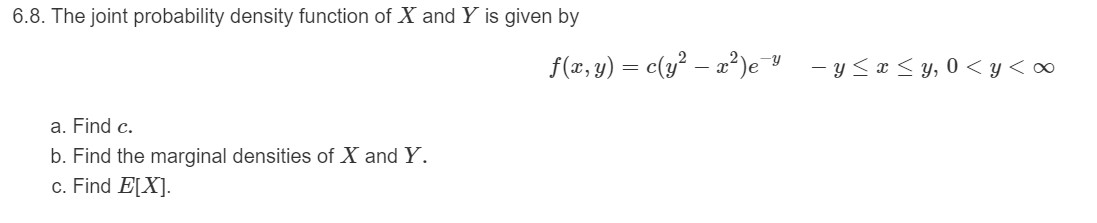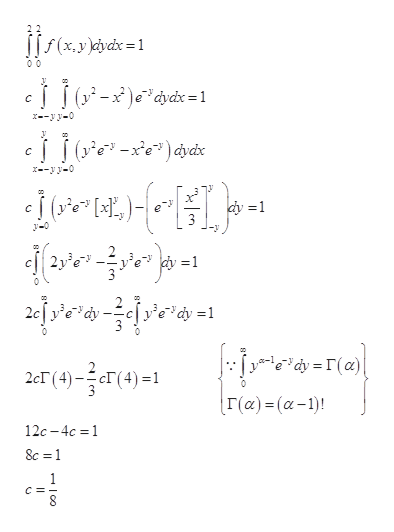# 6.8. The joint probability density function of X and Y is given byf(x, y) = c(y² – a?)e-y- y

Question
1 views

2help_outlineImage Transcriptionclose6.8. The joint probability density function of X and Y is given by f(x, y) = c(y² – a?)e -y - y
check_circle

Step 1

2.

The joint probability density function of X and Y is given by,

Step 2

a).

The value of c is calculated below:help_outlineImage Transcriptionclosef(x,y cydx=1 00 ر(آأء-x)c*dbdx = 1 C C 2y =1 edy (a) 24T(4)-1 2cГ (4) Γ (α) - (α -1)! 12c-4c 1 8c =1 fullscreen
Step 3

b).

The marginal density of X can be...

### Want to see the full answer?

See Solution

#### Want to see this answer and more?

Solutions are written by subject experts who are available 24/7. Questions are typically answered within 1 hour.*

See Solution
*Response times may vary by subject and question.
Tagged in
MathStatistics

### Other